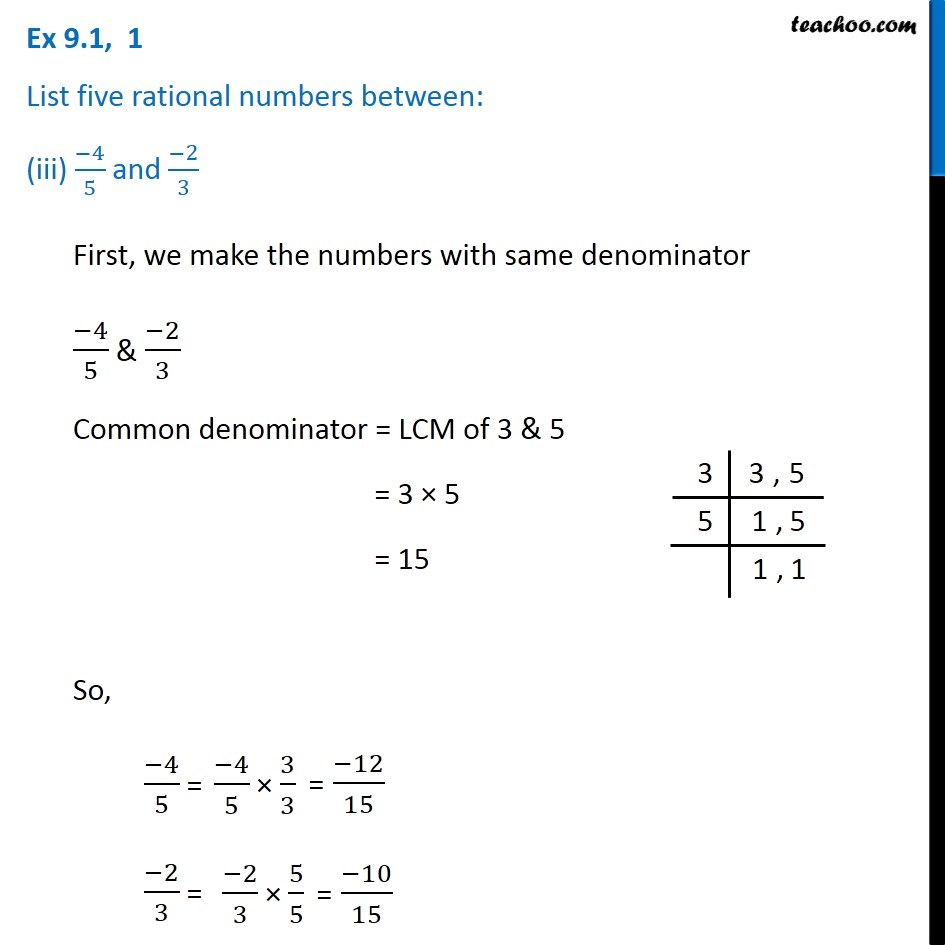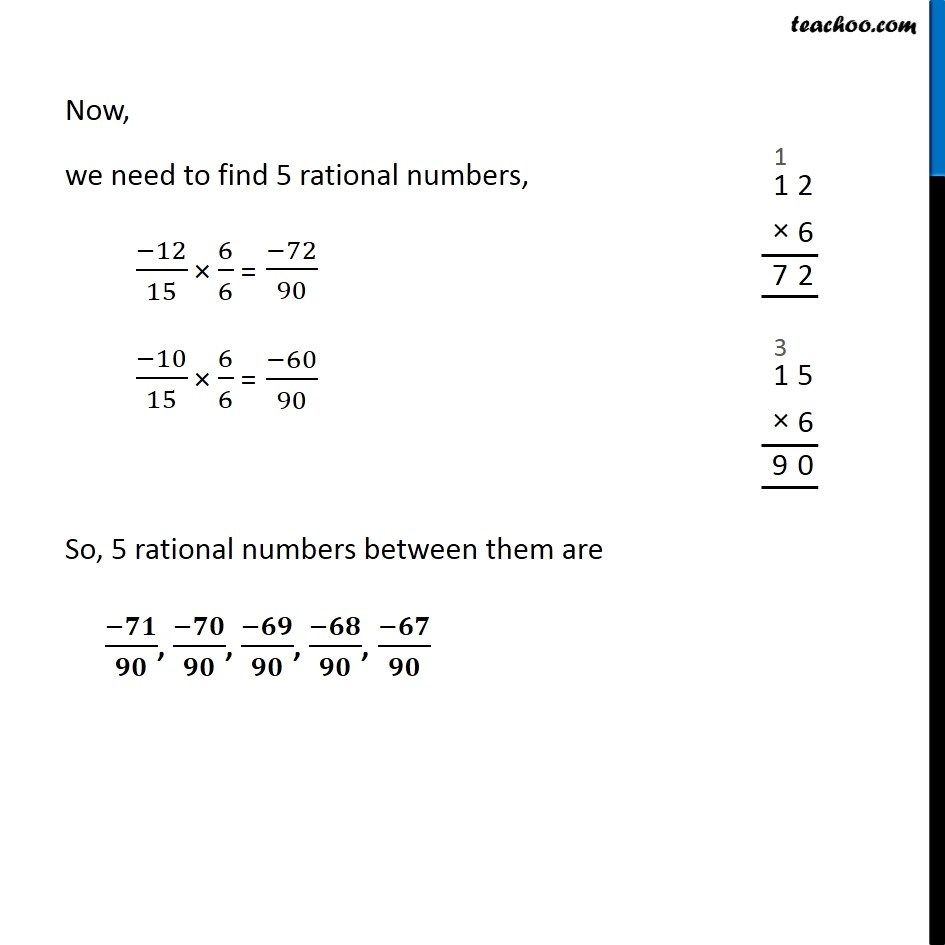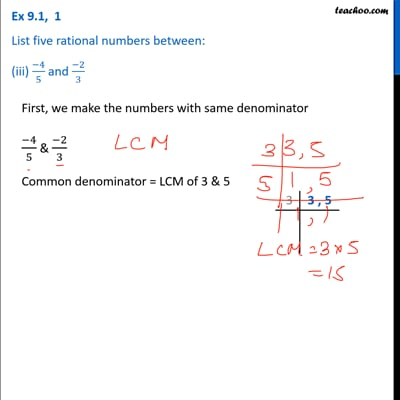Ex 9.1

Chapter 9 Class 7 Rational Numbers
Serial order wiseThis video is only available for Teachoo black users

Get live Maths 1-on-1 Classs - Class 6 to 12

### Transcript

Ex 9.1, 1 List five rational numbers between: (iii) (−4)/5 and (−2)/3 First, we make the numbers with same denominator (−4)/5 & (−2)/3 Common denominator = LCM of 3 & 5 = 3 × 5 = 15 So, (−4)/5 =(−4)/5 × 3/3 = (−12)/15 (−2)/3 = (−2)/3 × 5/5 = (−10)/15 Now, we need to find 5 rational numbers, (−12)/15 × 6/6 =(−72)/90 (−10)/15 × 6/6 =(−60)/90 So, 5 rational numbers between them are (−𝟕𝟏)/𝟗𝟎, (−𝟕𝟎)/𝟗𝟎, (−𝟔𝟗)/𝟗𝟎, (−𝟔𝟖)/𝟗𝟎, (−𝟔𝟕)/𝟗𝟎